# Probing the BOS1901 with an oscilloscope

Oscilloscopes are very useful instruments when building applications with the BOS1901. Probing the voltage generated on BOS1901 output can convey a lot of information when used properly. However, when connected incorrectly, bad things are bound to happen.

This application note explains how to properly connect and setup an oscilloscope to probe the BOS1901 high-voltage output signals and what to avoid.

# 1 BOS1901 Basics

The BOS1901 is a single-chip piezo actuator driver with energy recovery, based on a patented CapDrive™ technology. It can drive actuators with up to 190 Vpp waveforms while operating from a 3 to 5.5 V supply voltage. Low power and small size make it ideal for a variety of applications requiring minimal power consumption and heat dissipation.

The 190 Vpp is achieved by alternating the voltage on the piezoelectric actuator from +95 V to -95 V.

When outputting +95 V, BOS1901 is in positive polarity. Pin OUT+ is set to VDD+95 V while pin OUT- is set to VDD. Thus the voltage difference seen by the actuator is +95 V.

When outputting -95 V, BOS1901 is in negative polarity. Pin OUT+ is set to VDD while pin OUT- is set to VDD+95 V. Thus the voltage difference seen by the actuator is -95 V.

In all situations, voltage on OUT+ and OUT- is driven between VDD and VDD+95 V. This means both piezo terminals are above the ground and considered active.

The figures below show how OUT+ and OUT- are behaving when the programmed voltage is positive (left) and negative (right). In both cases, both OUT+ and OUT- output a positive voltage. It is the voltage difference between both terminals that changes polarity.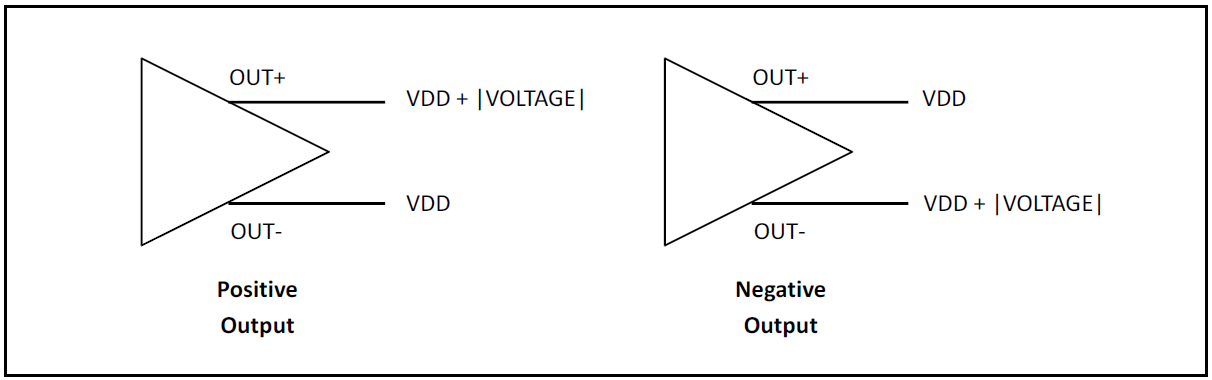Figure 1 OUT+ and OUT- voltage level when programming a positive (left) and a negative (right) voltage

# 2 What to Avoid

Before showing the proper way to connect the oscilloscope probes, let’s first start by showing how not to connect them.

Never connect an oscilloscope probe ground connection to one of the actuator terminals.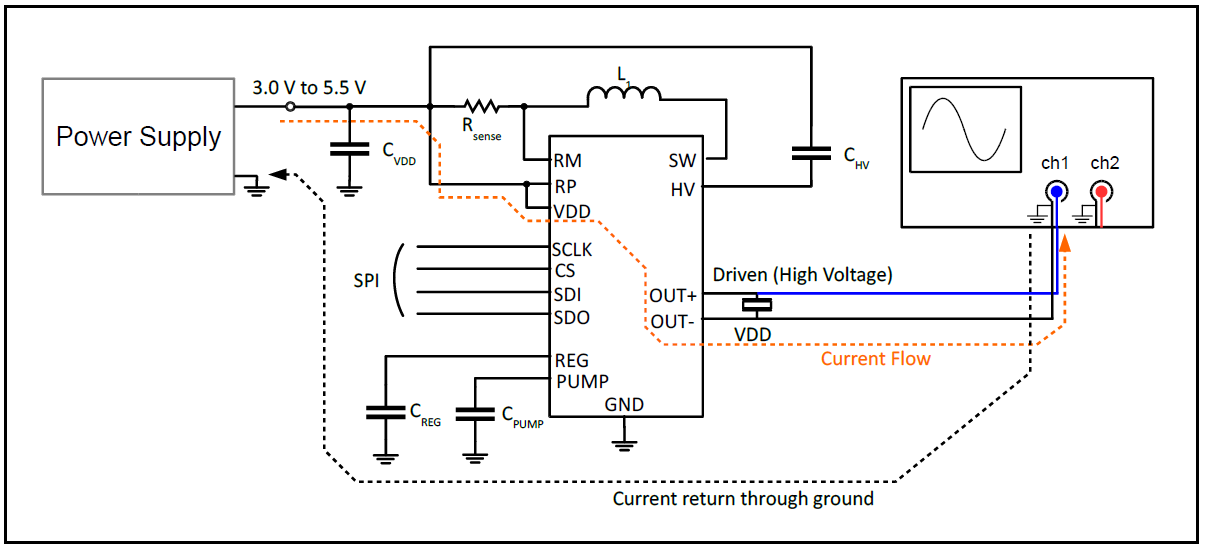Figure 1 Incorrect oscilloscope probe connection to BOS1901

Connecting the oscilloscope probe like this creates a short-circuit between VDD and ground. An oscilloscope probe ground is shorted with the instrument ground connection. The current flows from the power supply into the IC VDD, then through OUT- which is set at VDD internally, then to the oscilloscope probe ground. The current loop is completed by the oscilloscope probe ground that is linked to the oscilloscope power ground internally. Through the power line, the power supply ground and oscilloscope grounds and linked.

This short between VDD and ground causes high current inside IC. This current is much higher than the normal operation current, creating a lot of heat dissipation in the IC, which can lead to IC breakdown, to the power supply failure, or worse, it might damage the oscilloscope.

# 3 Proper Oscilloscope Connection

The following figure shows the proper connection between an oscilloscope and a BOS1901.

Two probes must be used, each on its channel on the oscilloscope:

a. The first probe (ch1) signal tip should be connected to the OUT+ pin (note this connection is labeled HV+ on a BOS1901-KIT terminal block).

b. The second probe (ch2) signal tip should be connected to the OUT- pin (labeled HV- on a BOS1901-KIT).

c. Each probe ground clip should be connected to the BOS1901 GND pin.

Each channel will display the voltage measured on the associated terminal. Since both signals are referenced to VDD, displaying the difference between channel 1 and 2 as a math channel is also useful. However, useful debugging information can still be obtained by inspecting the individual channels.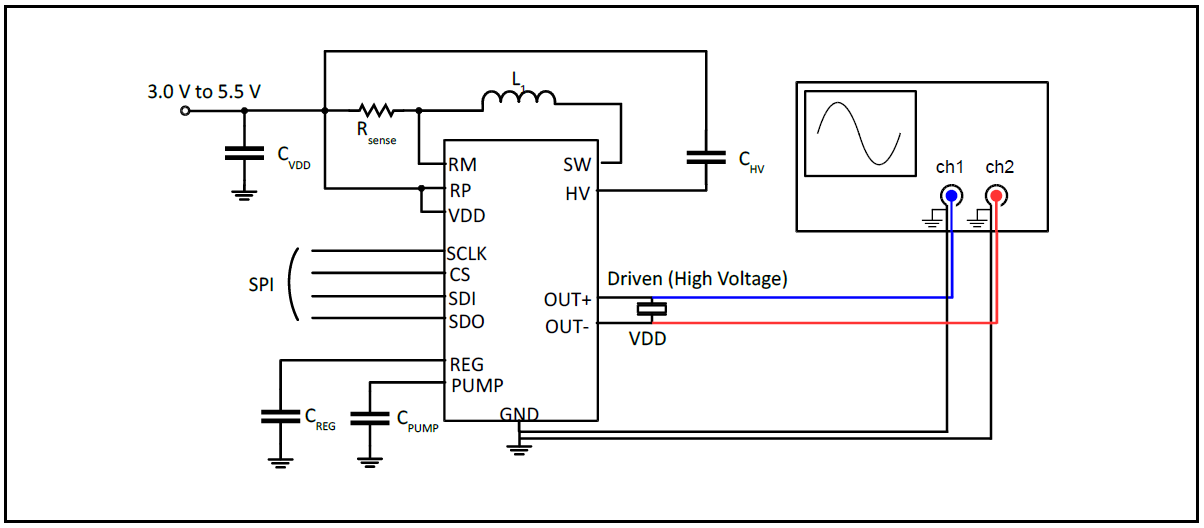Figure 2 Correct oscilloscope probe connection to BOS1901

An alternate method is to use a differential high-voltage probe. This allows to connect both terminals of the probe directly to OUT+ and OUT- and then read the voltage between these nodes directly on a single channel, thereby enabling processing such as FFT on the voltage applied to the piezoelectric actuator. Note however that doing so loses the DC reference voltage component on each node, which is useful in some debug cases.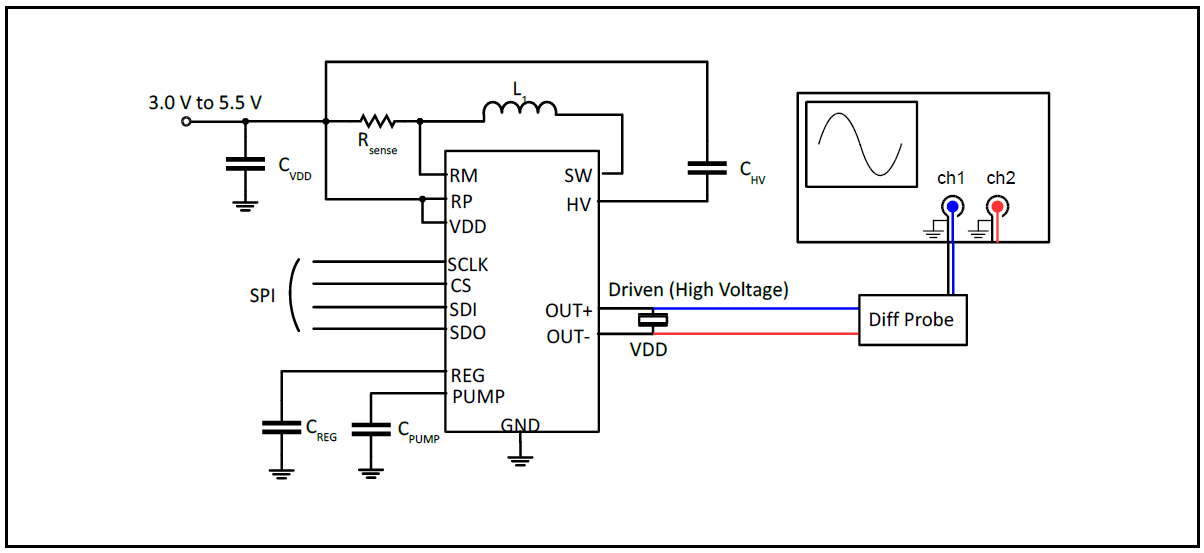Figure 3 Oscilloscope connection to BOS1901 using a differential probe

# 4 Oscilloscope Settings

When probing a BOS1901, the voltages measured may reach slightly above 100 V. Use oscilloscope probes that are fit for such voltage levels. Typically, a 10x probes will allow for such measurement.

Set both scope channels vertical scale to DC mode, 100 V range. Setting to AC mode is possible, but doing so loses the DC offset information, and since these are large signals, the offset is a useful information when debugging.

Set the time scale accordingly to the features you wish to analyze. For example, a 100 Hz signal will have a 10 ms period. Setting the time scale between 2 and 5 ms/div is appropriate to see a few cycles over a single capture.

The oscilloscope sampling rate is not required to be very high since the signals are generally not higher than a few hundred hertz. A 100 kHz sampling rate is generally enough in most cases.

# 5 Scope Capture Examples

The following figure shows a scope capture of a 100 Hz sine wave using the full range of operation. The blue curve is the positive output (OUT+), the red curve is the negative output (OUT-) and the purple curve is the differential voltage (OUT+ minus OUT-). Both signals only go down to the VDD supply level of 5 V while the difference varies between -95 and +95 V.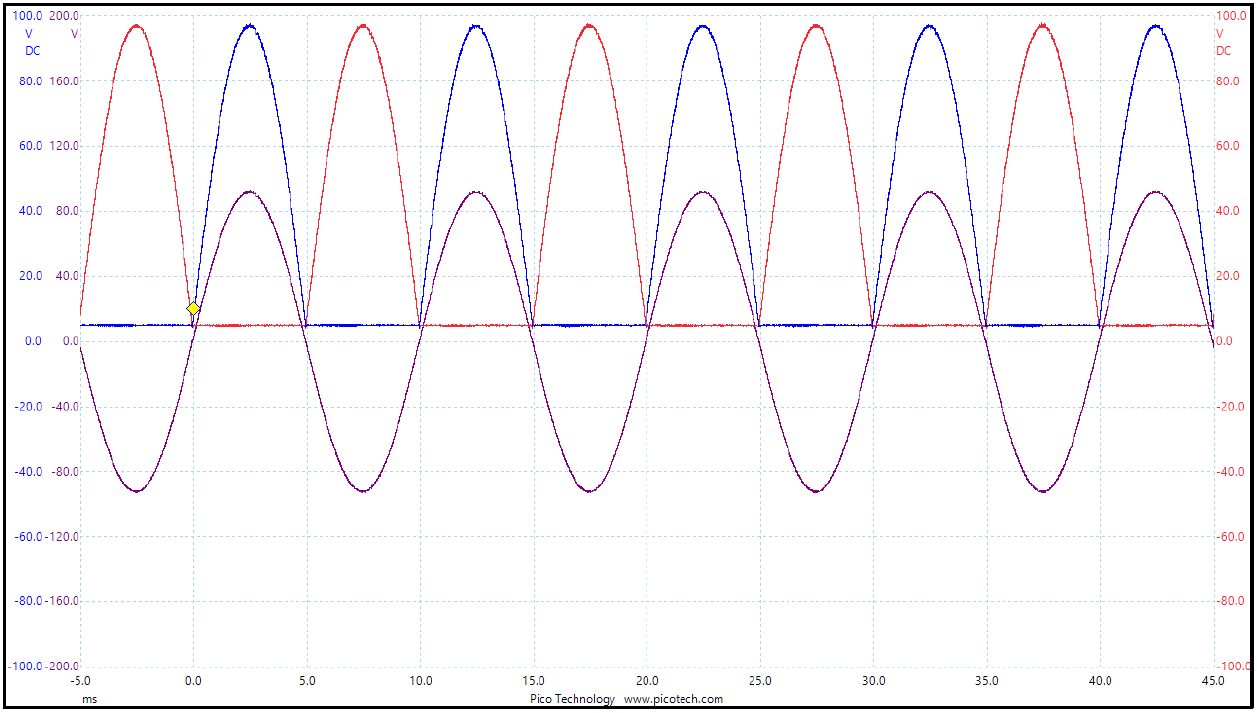Figure 4 ±95 V sine wave

The following figure shows a scope capture of a 100 Hz sine wave using only positive polarity between 0 and 60 V. Here only the positive terminal varies between 5 V and 65 V while the negative terminal is constant at 5 V. The difference is a 60 V amplitude sine waveform.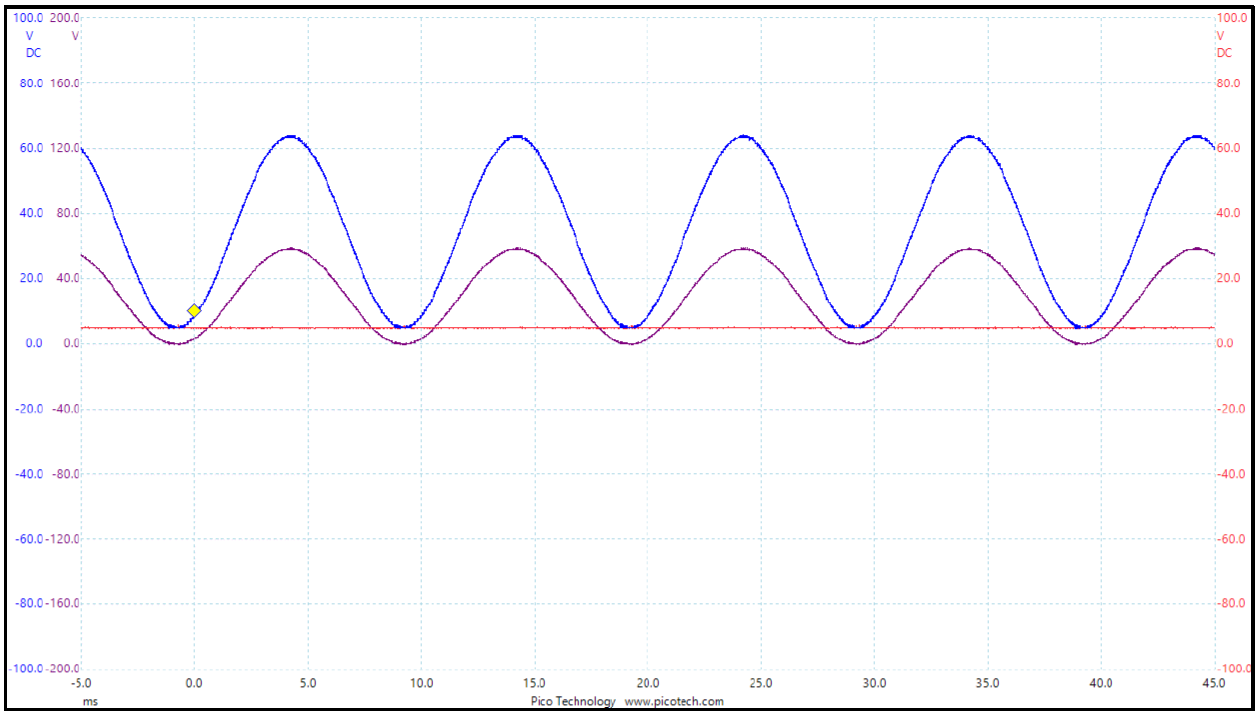Figure 5 0 to 60 V sine wave

# 6 Conclusion

Oscilloscopes are very useful instruments either when building or debugging application code. However, they must be correctly connected and set up to avoid damage to the prototype and the instrument.

# 7 Related Parts

 Part Number Description 1 BOS1901 BOS1901 Piezo Haptic Driver with Digital Front End